Fitting and Comparing Candidate Models

Fitting a Regression Model

On the Model tab, drag a Regression node to your diagram workspace. Connect the Interactive Binning node to the Regression (3) node.
In the Properties Panel, locate the Model Selection subgroup. These properties determine whether and which type of model of variable selection is performed when generating the model. You can choose from the following variable selection techniques:
• Backward — Variable selection begins with all candidate effects in the model and systematically removes effects that are not significantly associated with the target. This continues until no other effect in the model meets the Stay Significance Level, or until the Stop Variable Number is met. This method is not recommended for binary or ordinal targets when there are many candidate effects or when there are many levels for some classification input variables.
• Forward — Variable selection begins with no candidate effects in the model and then systematically adds effects that are significantly associated with the target. This proceeds until none of the remaining effects meet the Entry Significance Level, or until the Stop Variable Number is met.
• Stepwise — Variable selection begins with no candidate effects in the model and then systematically adds effects that are significantly associated with the target. After an effect is added to the model, it can be removed if it is deemed that the effect is no longer significantly associated with the target.
• None — No model selection is performed and all candidate effects are included in the final model.
For this example, set the value of the Selection Model property to Forward. Set the value of the Use Selection Defaults property to No. After you do this, notice that the Selection Options property becomes available.
Click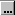next to the Selection Options property. The Selection Options window contains the Entry Significance Level, Stay Significance Level, Start Variable Number, and Stop Variable Number properties that were just mentioned. These properties enable you to control the significance level required for an effect to remain in or be removed from a model and the number of effects in a model. For Backward selection, the Stop Variable Number determines the minimum number of effects. For Forward selection, the Stop Variable Number determines the maximum number of effects.
The Maximum Number of Steps property enables you to set the maximum number of steps before the Stepwise method stops. The default value is twice the number of effects in the model.
The Hierarchy Effects property determines which variables are subject to effect hierarchies. Specify Class if you want to include just the classification variables and All if you want to use both the classification and interval variables.
Model hierarchy refers to the requirement that for any effect in the model, all effects that it contains must also be in the model. For example, in order for the interaction A*B to be in the model, the main effects A and B must also be in the model.
For this example, set the value of the Stay Significance Level to `0.025`. Set the value of the Start Variable Number to `10`. This ensures that at least 10 effects will be used by the regression model. Use the default settings for every other property.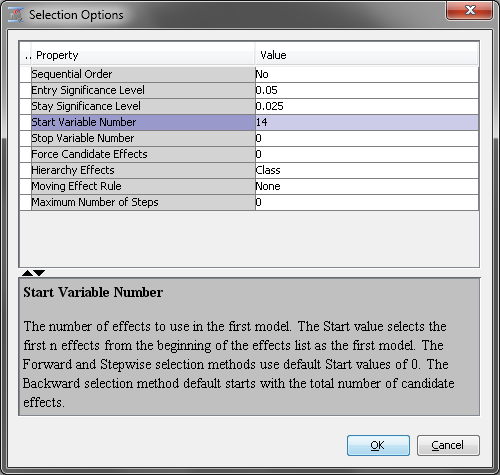Click OK.

Evaluating the Models

On the Assess tab, drag a Model Comparison node to your diagram workspace. Connect the Regression, Regression (2), and Regression (3) nodes to the Model Comparison node.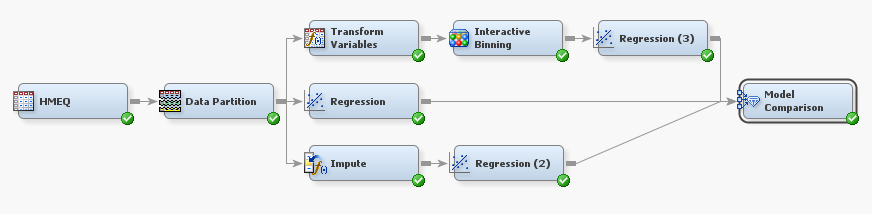Right-click the Model Comparison node and click Run. In the Confirmation window click Yes. In the Run Status window click Results.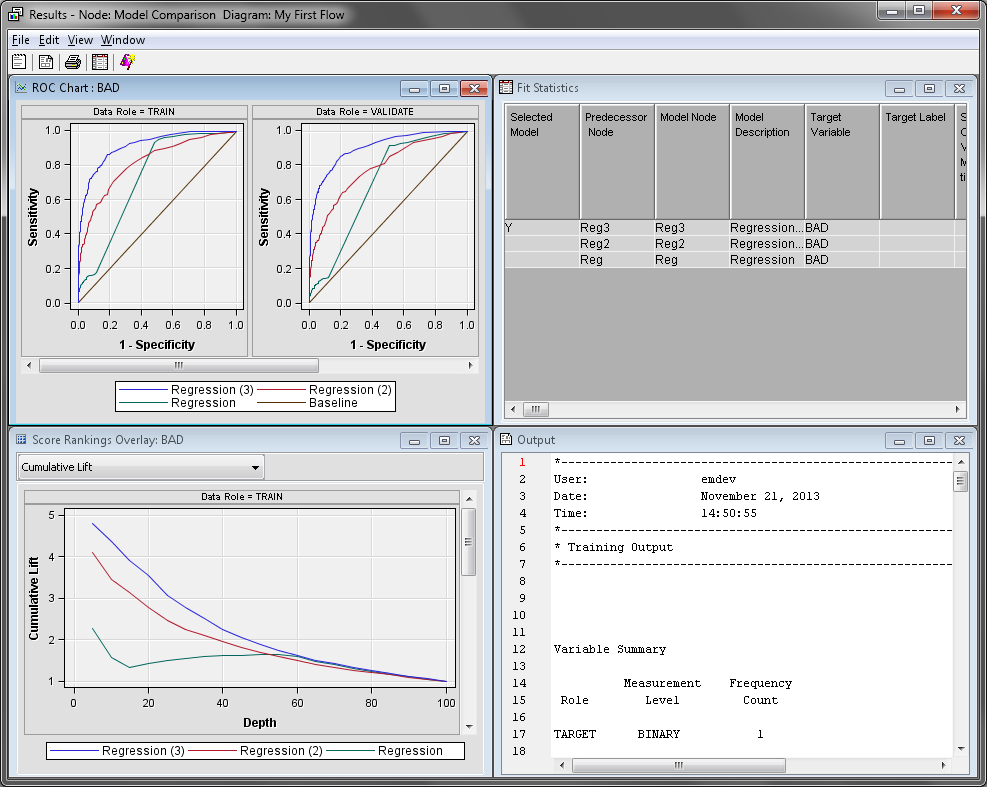The Model Comparison node Results window displays a lift chart that contains all three models. Maximize the Score Rankings Overlay window. On the drop-down menu, click Cumulative % Response.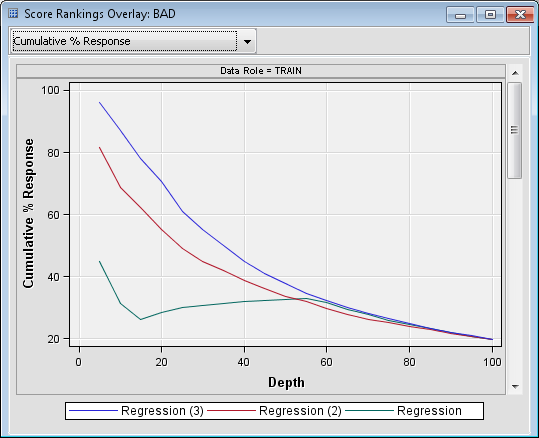Recall that this chart groups individuals based on the predicted probability of response, and then plots the percentage of respondents. Notice that the model created by the Regression (3) node is better at almost every depth.
The model created in the Regression (2) node was created with no effect selection, because it used the default settings of the Regression node. Therefore, that model contains every effect.
Minimize the Score Rankings Overlay window.
Maximize the Output window. Scroll down until you find the Fit Statistics Table. This table lists all of the calculated assessment statistics for each model that was compared. Statistics are computed separately for each partition of the input data. These statistics are also displayed in the Fit Statistics window.
Explore the rest of the charts and tables in the Results window. Close the Results window when you are finished.

Fitting a Default Decision Tree

Now that you have modeled the input data with three different regression models, you want to fit a tree model to determine whether it will make better predictions. On the Model tab, drag a Decision Tree node to your diagram workspace. Connect the Data Partition node to the Decision Tree node. Connect the Decision Tree node to the Model Comparison node.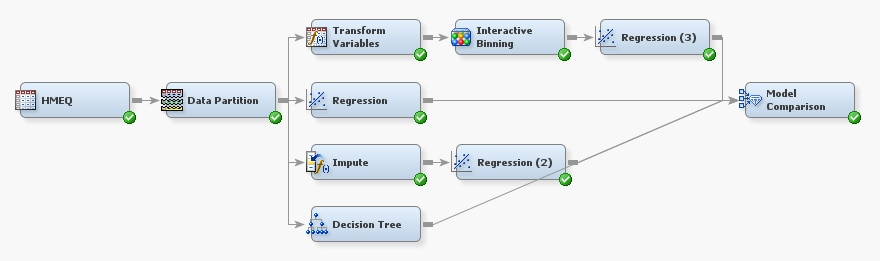Tree models handle missing values differently than regression models, so you can directly connect the Data Partition node to the Decision Tree node.
Monotonic transformations of interval numeric variables are not likely to improve the tree model because the tree groups numeric variables into bins. In fact, the tree model might perform worse if you connect it after you group the input variables. The bins created in the Interactive Binning node reduce the number of splits that the tree model can consider, unless you include both the original and binned variables.
Right-click the Model Comparison node and click Run. In the Confirmation window, click Yes. In the Run Status window, click Results.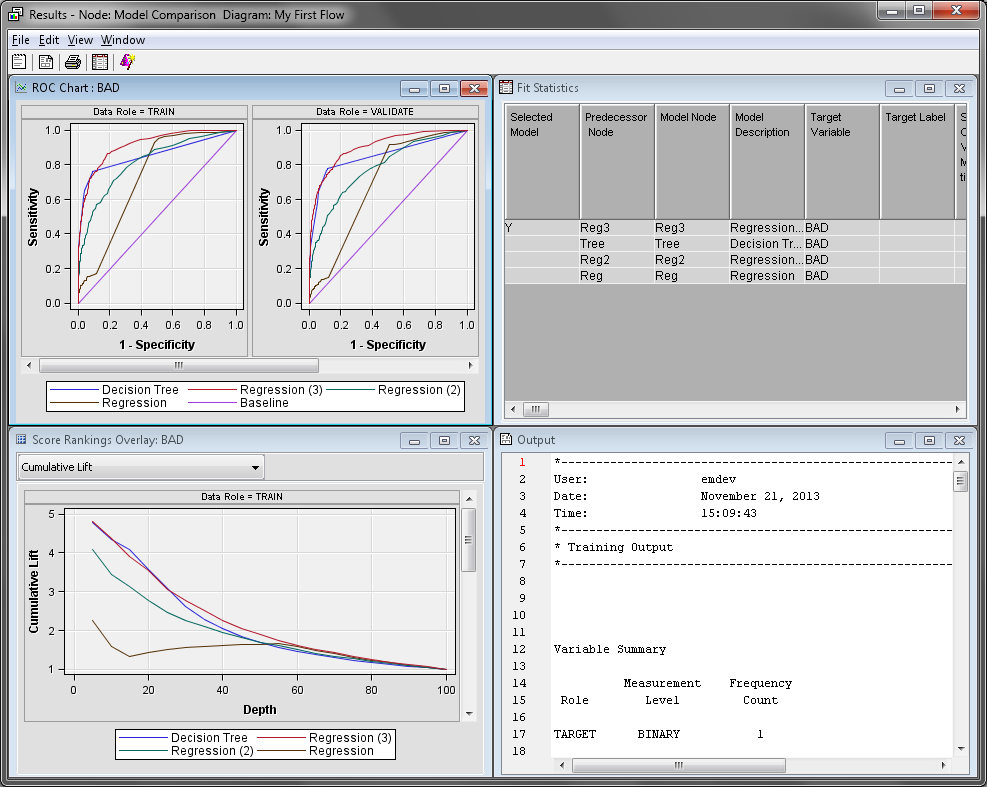In the Score Rankings Overlay window, notice that Cumulative % Response graphs for the Decision Tree model and the Regression (3) are fairly similar. However, the Regression (3) model is better as the depth increases.
The Fit Statistics window confirms that the Regression (3) model is the better model based on the selection criteria of the Model Comparison node.
Explore the rest of the charts and tables in the Results window. Close the Results window when you are finished.

Exploring the Tree Model Results

In your diagram workspace, right-click the Decision Tree node and click Results.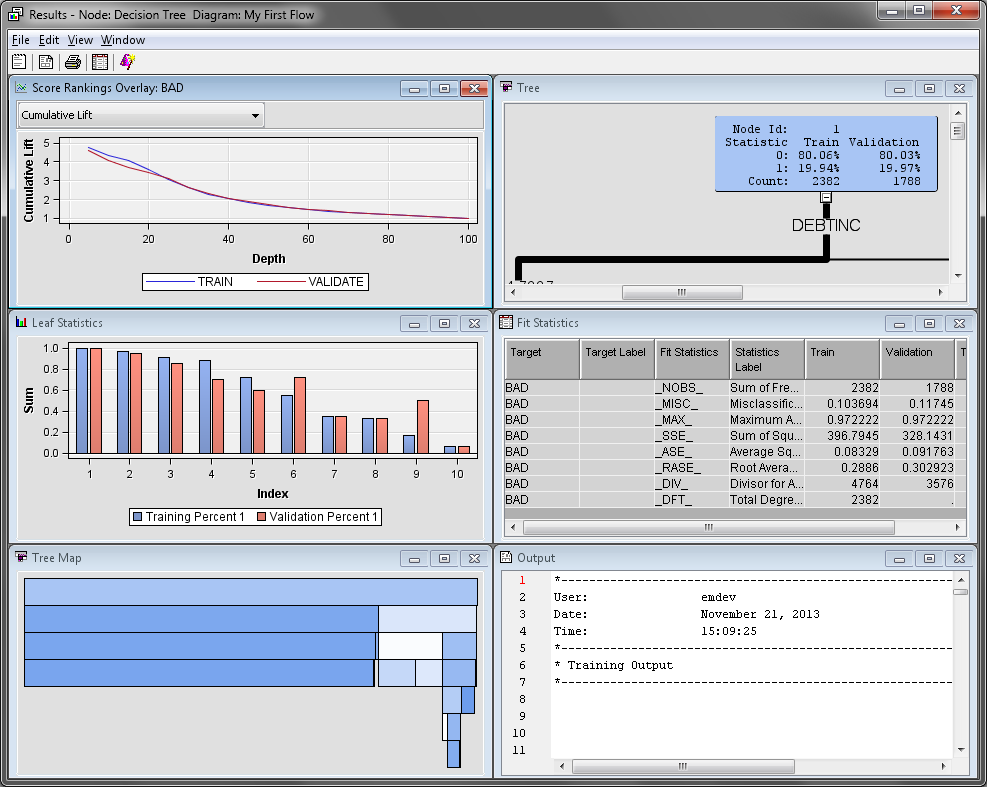The Tree Map and Tree windows present two different views of the decision tree. The Tree window displays a standard decision tree, where the node splits are provided on the links between the nodes. Inside the nodes, you can find the node ID, the training and validation classification rates, and the number of observations in the node for each partition. The Tree Map window uses the width of each node to visually display the percentage of observations in each node on that row. Selecting a node in one window selects the corresponding node in the other window.
The color of each node in the decision tree is based on the target variable. The more observations where BAD=1, the darker the node color is.
The Leaf Statistics window displays the training and validation classification rates for each leaf node in the tree model. Leaf nodes are terminal nodes in the decision tree. Select a bar in the Leaf Statistics window to select the corresponding leaf node in the Tree and Tree Map windows.
The Fit Statistics window displays several computed statistics for the tree model. These are computed for all partitions of the training data.
Explore the rest of the charts and tables in the Results window. Close the Results window when you are finished.

Fitting a Default Neural Network

Next, you want to compare how well a neural network model compares to the regression and tree models. On the Model tab, drag a Neural Network node to your diagram workspace. Connect the Interactive Binning node to the Neural Network node. Connect the Neural Network node to the Model Comparison node.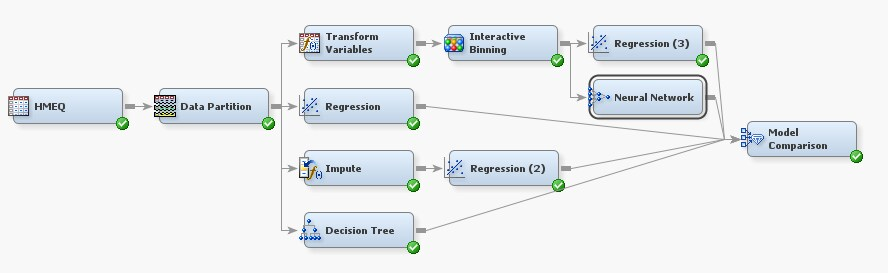By default, the Neural Network node creates a multilayer perceptron (MLP) model with no direct connections, and the number of hidden layers is data-dependent.
Right-click the Neural Network node and click Run. In the Confirmation window, click Yes. Click Results in the Run Status window.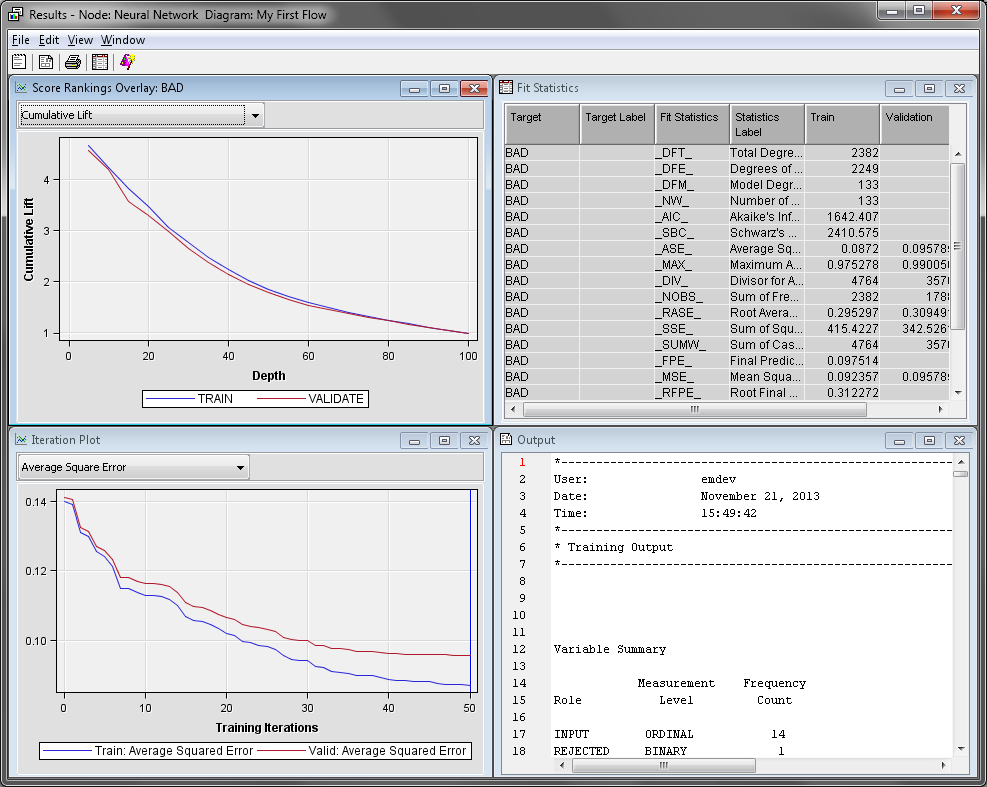The Fit Statistics window displays various computed statistics for the neural network model. The Iteration Plot window displays various statistics that were computed at each iteration during the creation of the neural network node.
Explore the rest of the charts and tables in the Results window. Close the Results window when you are finished.
In the diagram workspace, select the Model Comparison node. Right-click the Model Comparison node and click Run. In the Confirmation window, click Yes. In the Run Status window, click Results.
Notice that the predictive power Neural Network model is very similar to the Decision Tree and Regression (3) models. However, both models are considered slightly better by the Model Comparison node based on the default assessment criteria. In the Fit Statistics window, the models are listed in order with best model being at the top of the table and the worst model at the bottom.
Explore the rest of the charts and tables in the Results window. Close the Results window when you are finished.

Investigating the Regression and Neural Network Models

The default neural network model does not perform any better than the regression model. If both models performed poorly compared to the decision tree model, poor performance might be due to how missing values are handled. The tree model directly handles observations with missing values while the regression and neural network models ignore those observations. This is why the Regression model is significantly worse than the Decision Tree model.
In the Neural Network and Regression (3) model, you transformed and binned the variables before creating the regression. When the variables were binned, classification variables were created and missing values were assigned to a level for each of the new classification variables.
The Regression (2) model uses imputation to handle missing values. The effect of this replacement is that you replace a missing value (perhaps an unusual value for the variable) with an imputed value (a typical value for the variable). Imputation can change an observation from being somewhat unusual with respect to a particular variable to very typical with respect to that variable.
For example, if someone applied for a loan and had a missing value for INCOME, the Impute node would replace that value with the mean value of INCOME by default. In practice, however, someone who has an average value for INCOME would often be evaluated differently than someone with a missing value for INCOME. Any models that follow the Impute node would not be able to distinguish the difference between these two applicants.
One solution to this problem is to create missing value indicator variables. These variables indicate whether an observation originally had a missing value before imputation is performed. The missing value indicators enable the Regression and Neural Network nodes to differentiate between observations that originally had missing values and observations with no missing values. The addition of missing value indicators can greatly improve a neural network or regression model.
Recall that you chose not to use indicator variables earlier. You are going to reverse that decision now. In your diagram workspace, select the Impute node. Locate the Type property in the Indicator Variables subgroup. Set the value of this property to Unique. Set the value of the Source property to Missing Values. Set the value of the Role property to Input.
In the diagram workspace, select the Model Comparison node. Right-click the Model Comparison node and click Run. In the Confirmation window, click Yes. In the Run Status window, click Results.
Notice that the Regression (2) model now outperforms the Neural Network model. In fact, it is the best model in the first decile of the training data.
Close the Results window.
In general, it is impossible to know which model will provide the best results when it is applied to new data. For this data, or any other, a good analyst considers many variations of each model and identifies the best model according to their own criteria. In this case, assume that Regression (3) is the selected model.